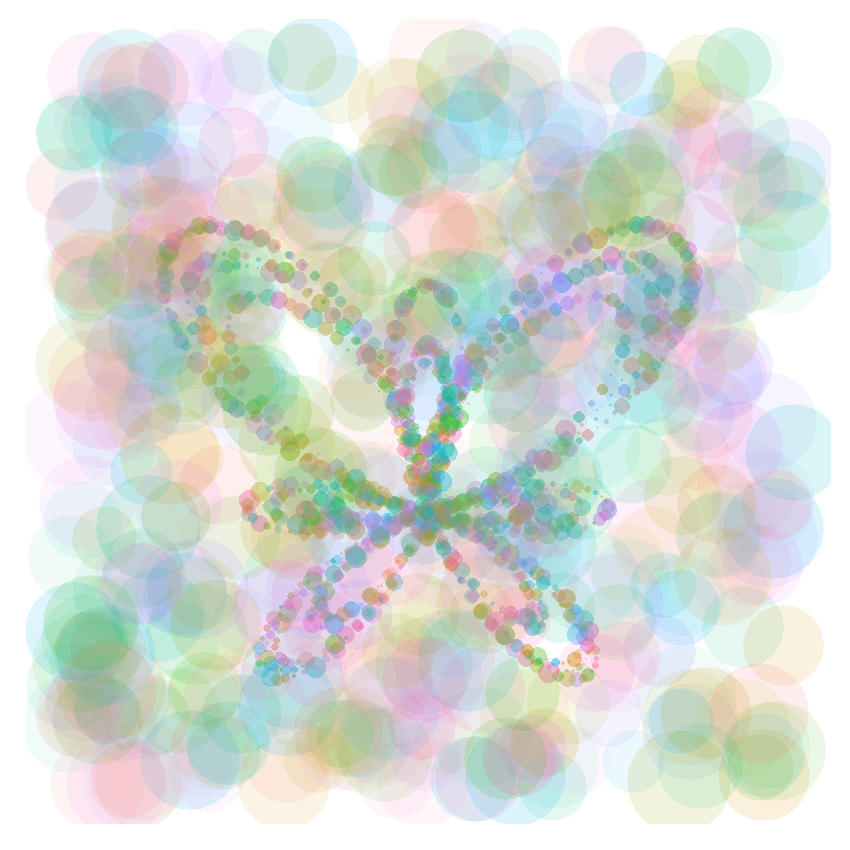# butteRfly

Float like a butterfly, sting like a bee (Muhammad Ali)

The Butterfly Curve was discovered by Temple H. Fay when he was in Southern University, Mississippi, and rapidly gained the attention of students and mathematicians because of its beautiful simmetry. Small dots of this plot are generated according to parametric equations of the Butterfly Curve. Big dots are randomdly distributed over the canvas:This is the code to create butterflies:

```library(ggplot2)
npoints=500
npointsb=1200
opt=theme(legend.position="none",
panel.background = element_blank(),
panel.grid = element_blank(),
axis.ticks=element_blank(),
axis.title=element_blank(),
axis.text =element_blank())
t=seq(0,10*pi,length=npointsb)
butterfly=data.frame(x=sin(t)*(exp(1)^cos(t)-2*cos(4*t)-(sin(t/12))^5), y=cos(t)*(exp(1)^cos(t)-2*cos(4*t)-(sin(t/12))^5), s=runif(npointsb, min=.1, max=10), f=factor(sample(1:10,npointsb,TRUE)), a=runif(npointsb,min=.1, max=.4))
points=data.frame(x=runif(npoints,-4,4), y=runif(npoints,-3,5), s=runif(npoints,min=30, max=50), f=factor(sample(1:10,npoints,TRUE)), a=runif(npoints,min=.05, max=.15))
data=rbind(butterfly, points)
ggplot(data, aes(x, y, colour=f))+geom_point(alpha=data\$a,size=data\$s)+opt
```